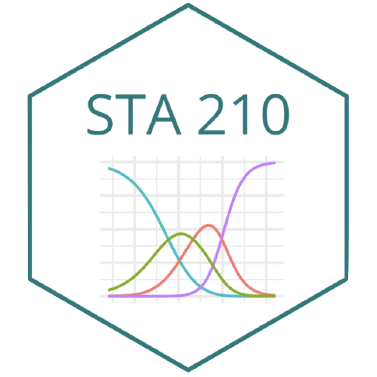# MultiLR: Predictive models (cont.)

STA 210 - Spring 2022

# Welcome

## Topics

• Unbalanced data
• Choosing the “final” model

## Computational setup

# load packages
library(tidyverse)
library(tidymodels)
library(knitr)
library(colorblindr)
library(themis)

# set default theme and larger font size for ggplot2
ggplot2::theme_set(ggplot2::theme_minimal(base_size = 16))

# From last time…

## Volcanoes

The data come from The Smithsonian Institution, via TidyTuesday.

volcano <- read_csv(here::here("slides", "data/volcano.csv"))
names(volcano)
  "volcano_number"           "volcano_name"
 "primary_volcano_type"     "last_eruption_year"
 "country"                  "region"
 "subregion"                "latitude"
 "longitude"                "elevation"
 "tectonic_settings"        "evidence_category"
 "major_rock_1"             "major_rock_2"
 "major_rock_3"             "major_rock_4"
 "major_rock_5"             "minor_rock_1"
 "minor_rock_2"             "minor_rock_3"
 "minor_rock_4"             "minor_rock_5"
 "population_within_5_km"   "population_within_10_km"
 "population_within_30_km"  "population_within_100_km"

## Data prep

volcano <- volcano %>%
mutate(
volcano_type = case_when(
str_detect(primary_volcano_type, "Stratovolcano") ~ "Stratovolcano",
str_detect(primary_volcano_type, "Shield") ~ "Shield",
TRUE ~ "Other"
),
volcano_type = fct_relevel(volcano_type, "Stratovolcano", "Shield", "Other")
) %>%
select(
volcano_type, latitude, longitude,
elevation, tectonic_settings, major_rock_1
) %>%
mutate(across(where(is.character), as_factor))

## Split into testing/training

set.seed(1234)

volcano_split <- initial_split(volcano)
volcano_train <- training(volcano_split)
volcano_test  <- testing(volcano_split)

## Specify a model

volcano_spec <- multinom_reg() %>%
set_engine("nnet")

volcano_spec
Multinomial Regression Model Specification (classification)

Computational engine: nnet 

## Create cross validation folds

set.seed(9876)

volcano_folds <- vfold_cv(volcano_train, v = 5)
volcano_folds
#  5-fold cross-validation
# A tibble: 5 × 2
splits            id
<list>            <chr>
1 <split [574/144]> Fold1
2 <split [574/144]> Fold2
3 <split [574/144]> Fold3
4 <split [575/143]> Fold4
5 <split [575/143]> Fold5

# Unbalanced data

## Unbalanced data

Remember that the observed volcano types are unbalanced:

volcano %>%
count(volcano_type)
# A tibble: 3 × 2
volcano_type      n
<fct>         <int>
1 Stratovolcano   461
2 Shield          118
3 Other           379

To address class unbalance, we generally use

• oversampling data from levels that are less prevalent in the data
• downsampling data from levels that are more prevalent in the data
• e.g., step_downsample(): Removes rows of a data set to make the occurrence of levels in a specific factor level equal.

## New recipe - oversample

volcano_rec3 <- recipe(volcano_type ~ ., data = volcano_train) %>%
step_other(tectonic_settings) %>%
step_other(major_rock_1) %>%
step_dummy(all_nominal_predictors()) %>%
step_zv(all_predictors()) %>%
step_center(all_predictors()) %>%
step_smote(volcano_type)

## New recipe - downsample

volcano_rec4 <- recipe(volcano_type ~ ., data = volcano_train) %>%
step_other(tectonic_settings) %>%
step_other(major_rock_1) %>%
step_dummy(all_nominal_predictors()) %>%
step_zv(all_predictors()) %>%
step_center(all_predictors()) %>%
step_downsample(volcano_type)

## New workflows

volcano_wflow3 <- workflow() %>%

volcano_wflow4 <- workflow() %>%
add_model(volcano_spec)

## Fit resamples

volcano_fit_rs3 <- volcano_wflow3 %>%
fit_resamples(
volcano_folds,
control = control_resamples(save_pred = TRUE)
)

volcano_fit_rs4 <- volcano_wflow4 %>%
fit_resamples(
volcano_folds,
control = control_resamples(save_pred = TRUE)
)

## Collect metrics

collect_metrics(volcano_fit_rs3)
# A tibble: 2 × 6
.metric  .estimator  mean     n std_err .config
<chr>    <chr>      <dbl> <int>   <dbl> <chr>
1 accuracy multiclass 0.517     5  0.0154 Preprocessor1_Model1
2 roc_auc  hand_till  0.693     5  0.0270 Preprocessor1_Model1
collect_metrics(volcano_fit_rs4)
# A tibble: 2 × 6
.metric  .estimator  mean     n std_err .config
<chr>    <chr>      <dbl> <int>   <dbl> <chr>
1 accuracy multiclass 0.485     5  0.0123 Preprocessor1_Model1
2 roc_auc  hand_till  0.675     5  0.0219 Preprocessor1_Model1

## ROC curves - oversampling

volcano_fit_rs3 %>%
collect_predictions() %>%
group_by(id) %>%
roc_curve(
truth = volcano_type,
.pred_Stratovolcano:.pred_Other
) %>%
autoplot()

## ROC curves - downsampling

volcano_fit_rs4 %>%
collect_predictions() %>%
group_by(id) %>%
roc_curve(
truth = volcano_type,
.pred_Stratovolcano:.pred_Other
) %>%
autoplot()

Can you think of any issues resulting from over/down sampling?

# Final model

## The “chosen” model

Let’s stick to the models without over/down sampling.

From the application exercise:

volcano_rec2 <- recipe(volcano_type ~ ., data = volcano_train) %>%
step_other(tectonic_settings) %>%
step_other(major_rock_1) %>%
step_dummy(all_nominal_predictors()) %>%
step_zv(all_predictors()) %>%
step_center(all_predictors())

volcano_wflow2 <- workflow() %>%
add_model(volcano_spec)

## Fitting the final model

final_fit <- last_fit(
volcano_wflow2,
split = volcano_split
)

collect_metrics(final_fit)
# A tibble: 2 × 4
.metric  .estimator .estimate .config
<chr>    <chr>          <dbl> <chr>
1 accuracy multiclass     0.629 Preprocessor1_Model1
2 roc_auc  hand_till      0.734 Preprocessor1_Model1

## Confusion matrix

collect_predictions(final_fit) %>%
conf_mat(volcano_type, .pred_class)
               Truth
Prediction      Stratovolcano Shield Other
Stratovolcano            96     13    38
Shield                    1      0     0
Other                    21     16    55

## Confusion matrix - visualized

collect_predictions(final_fit) %>%
conf_mat(volcano_type, .pred_class) %>%
autoplot()

## ROC curve

collect_predictions(final_fit) %>%
roc_curve(truth = volcano_type, .pred_Stratovolcano:.pred_Other) %>%
autoplot()

## Prediction

final_fitted <- extract_workflow(final_fit)

new_volcano <- tibble(
latitude = 35.9940,
longitude = -78.8986,
elevation = 404,
tectonic_settings = "Subduction zone / Continental crust (>25 km)",
major_rock_1 = "Andesite / Basaltic Andesite"
)

predict(
final_fitted,
new_volcano,
type = "prob"
)
# A tibble: 3 × 1
.pred_value
<dbl>
1      0.381
2      0.0379
3      0.581 

## Acknowledgements

Inspired by

• https://juliasilge.com/blog/multinomial-volcano-eruptions/
• https://juliasilge.com/blog/nber-papers/